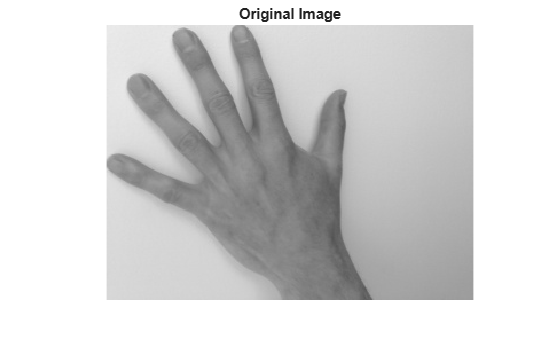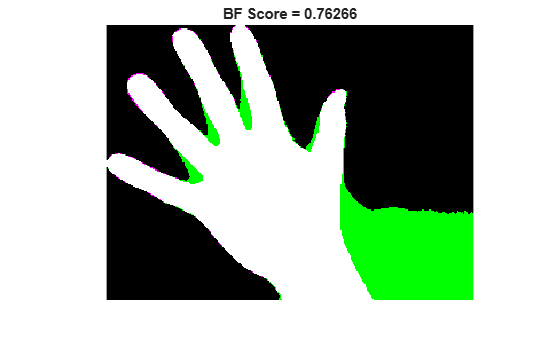Main Content

# bfscore

Contour matching score for image segmentation

## Syntax

``score = bfscore(prediction,groundTruth)``
``[score,precision,recall] = bfscore(prediction,groundTruth)``
``[___] = bfscore(prediction,groundTruth,threshold)``

## Description

example

````score = bfscore(prediction,groundTruth)` computes the BF (Boundary F1) contour matching score between the predicted segmentation in `prediction` and the true segmentation in `groundTruth`. `prediction` and `groundTruth` can be a pair of logical arrays for binary segmentation, or a pair of label or categorical arrays for multiclass segmentation.```
````[score,precision,recall] = bfscore(prediction,groundTruth)` also returns the precision and recall values for the `prediction` image compared to the `groundTruth` image.```
````[___] = bfscore(prediction,groundTruth,threshold)` computes the BF score using a specified threshold as the distance error tolerance, to decide whether a boundary point has a match or not.```

## Examples

collapse all

Read an image with an object to segment. Convert the image to grayscale, and display the result.

```A = imread('hands1.jpg'); I = im2gray(A); figure imshow(I) title('Original Image')```Use the active contours (snakes) method to segment the hand.

```mask = false(size(I)); mask(25:end-25,25:end-25) = true; BW = activecontour(I, mask, 300);```

Read the ground truth segmentation.

`BW_groundTruth = imread('hands1-mask.png');`

Compute the BF score of the active contours segmentation against the ground truth.

`similarity = bfscore(BW, BW_groundTruth);`

Display the masks on top of each other. Colors indicate differences in the masks.

```figure imshowpair(BW, BW_groundTruth) title(['BF Score = ' num2str(similarity)])```This example shows how to segment an image into multiple regions. The example then computes the BF score for each region.

Read an image with several regions to segment.

`RGB = imread('yellowlily.jpg');`

Create scribbles for three regions that distinguish their typical color characteristics. The first region classifies the yellow flower. The second region classifies the green stem and leaves. The last region classifies the brown dirt in two separate patches of the image. Regions are specified by a 4-element vector, whose elements indicate the x- and y-coordinate of the upper left corner of the ROI, the width of the ROI, and the height of the ROI.

```region1 = [350 700 425 120]; % [x y w h] format BW1 = false(size(RGB,1),size(RGB,2)); BW1(region1(2):region1(2)+region1(4),region1(1):region1(1)+region1(3)) = true; region2 = [800 1124 120 230]; BW2 = false(size(RGB,1),size(RGB,2)); BW2(region2(2):region2(2)+region2(4),region2(1):region2(1)+region2(3)) = true; region3 = [20 1320 480 200; 1010 290 180 240]; BW3 = false(size(RGB,1),size(RGB,2)); BW3(region3(1,2):region3(1,2)+region3(1,4),region3(1,1):region3(1,1)+region3(1,3)) = true; BW3(region3(2,2):region3(2,2)+region3(2,4),region3(2,1):region3(2,1)+region3(2,3)) = true;```

Display the seed regions on top of the image.

```figure imshow(RGB) hold on visboundaries(BW1,'Color','r'); visboundaries(BW2,'Color','g'); visboundaries(BW3,'Color','b'); title('Seed regions')```Segment the image into three regions using geodesic distance-based color segmentation.

`L = imseggeodesic(RGB,BW1,BW2,BW3,'AdaptiveChannelWeighting',true);`

Load a ground truth segmentation of the image.

`L_groundTruth = double(imread('yellowlily-segmented.png'));`

Visually compare the segmentation results with the ground truth.

```figure imshowpair(label2rgb(L),label2rgb(L_groundTruth),'montage') title('Comparison of Segmentation Results (Left) and Ground Truth (Right)')```Compute the BF score for each segmented region.

`similarity = bfscore(L, L_groundTruth)`
```similarity = 3×1 0.7992 0.5333 0.7466 ```

The BF score is noticeably smaller for the second region. This result is consistent with the visual comparison of the segmentation results, which erroneously classifies the dirt in the lower right corner of the image as leaves.

## Input Arguments

collapse all

Predicted segmentation, specified as a 2-D or 3-D logical, numeric, or `categorical` array. If `prediction` is a numeric array, then it represents a label array and must contain nonnegative integers of data type `double`.

Data Types: `logical` | `double` | `categorical`

Ground truth segmentation, specified as a 2-D or 3-D logical, numeric, or `categorical` array of the same size and data type as `prediction`. If `groundTruth` is a numeric array, then it represents a label array and must contain nonnegative integers of data type `double`.

Data Types: `logical` | `double` | `categorical`

Distance error tolerance threshold in pixels, specified as a positive scalar. The threshold determines whether a boundary point has a match or not. If `threshold` is not specified, then the default value is 0.75% of the length of the image diagonal.

Example: `3`

Data Types: `double`

## Output Arguments

collapse all

BF score, returned as a numeric scalar or vector with values in the range [0, 1]. A `score` of 1 means that the contours of objects in the corresponding class in `prediction` and `groundTruth` are a perfect match. If the input arrays are:

• logical arrays, `score` is a scalar and represents the BF score of the foreground.

• label or categorical arrays, `score` is a vector. The first coefficient in `score` is the BF score for the first foreground class, the second coefficient is the score for the second foreground class, and so on.

Precision, returned as a numeric scalar or numeric vector with values in the range [0, 1]. Each element indicates the precision of object contours in the corresponding foreground class.

Precision is the ratio of the number of points on the boundary of the predicted segmentation that are close enough to the boundary of the ground truth segmentation to the length of the predicted boundary. In other words, precision is the fraction of detections that are true positives rather than false positives.

Recall, returned as a numeric scalar or numeric vector with values in the range [0, 1]. Each element indicates the recall of object contours in the corresponding foreground class.

Recall is the ratio of the number of points on the boundary of the ground truth segmentation that are close enough to the boundary of the predicted segmentation to the length of the ground truth boundary. In other words, recall is the fraction of true positives that are detected rather than missed.

## More About

collapse all

### BF (Boundary F1) Score

The BF score measures how close the predicted boundary of an object matches the ground truth boundary.

The BF score is defined as the harmonic mean (F1-measure) of the `precision` and `recall` values with a distance error tolerance to decide whether a point on the predicted boundary has a match on the ground truth boundary or not.

`score` = 2 * `precision` * `recall` / (`recall` + `precision`)

 Csurka, G., D. Larlus, and F. Perronnin. "What is a good evaluation measure for semantic segmentation?" Proceedings of the British Machine Vision Conference, 2013, pp. 32.1-32.11.

Download ebook